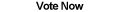Home Articles Components Code Snippets Links# C# Greatest Common Denominator Euclidean Algorithm## Greatest Common Denominator

The greatest common denominator, or GCD, between two numbers is the greatest integer that divides both given numbers. Writing a C# program that can calculate the greatest common denominator (GCD) of large numbers quickily would be very useful.

The first idea that comes to mind is perhaps to write a for loop to check all numbers that are less than the pair of numbers to find the GCD. However there is a more efficient way using the Euclidean Algorithm.

## Euclidean Algorithm

The Euclidean Algorithm is pretty straight forward: given two numbers, repeatedly replace the larger number with the greater number mod the lesser number. Keep repeating the step until one of the two numbers reaches zero, the other number will then be the greatest common denominator (or greatest common divisor), the GCD.

## Recursive

The Euclidean Algorithm lends itself to a recursive C# function (a function that calls itself):

```public int GCDRecursive(int a, int b)
{
if (a == 0)
return b;
if (b == 0)
return a;

if (a > b)
return GCDRecursive(a % b, b);
else
return GCDRecursive(a, b % a);
}```

Looking over the code it does exactly what the Euclidean Algorithm describes: repeatedly calls itself until one of the two numbers reaches zero.

## Non-Recursive

For a variety of reasons, you might want a C# function that is non-recursive. It is not as elegant or simple to understand, but it can be written:

```public int GCD(int a, int b)
{
while (a != 0 && b != 0)
{
if (a > b)
a %= b;
else
b %= a;
}

if (a == 0)
return b;
else
return a;
}```

## Speed

One reason for using the non-recursive Euclidean Algorithm function over the recursive one to find the greatest common denominator is for speed. .NET functions that call themselves suffer a slight performance impact. For example, some rough tests using the SpeedTester C# Class showed a 34% increase in speed by using the non-recursive GCD function.

Back to C# Article List

Other C# Articles